# Light Reflection and Refraction Class 10 Extra Questions with Answers Science Chapter 10

In this page, you can find CBSE Class 10 Science Chapter 10 Extra Questions and Answers Light Reflection and Refraction Pdf free download, NCERT Extra Questions for Class 10 Science will make your practice complete.

## Class 10 Science Chapter 10 Extra Questions and Answers Light Reflection and Refraction

Extra Questions for Class 10 Science Chapter 10 Light Reflection and Refraction with Answers Solutions

### Light Reflection and Refraction Extra Questions Very Short Answer Type

Question 1.
What is light?
Light is a form of energy which gives us the sensation of sight or vision.

Question 2.
What is a ray of light?
The path along which light travels is called a ray of light.

Question 3.
What is a beam of light?
A group of light rays originating from a source and travelling in some definite direction is known as a beam of light.

Question 4.
Define the term principal axis of a spherical mirror.
The principal axis of a spherical mirror is the line joining the pole and the centre of curvature of a spherical mirror.

Question 5.
Name a communication device which uses light for its working.
Optical fibres, which transmit many telephonic messages at the same time.

Question 6.
What is the angle of reflection when a ray of light falls normally on a plane mirror?
The angle of reflection is 0°.

Question 7.
What kind of image can be obtained on the screen?
Real image.

Question 8.
What type of image is formed:
(i) in a plane mirror, and
(ii) on a cinema screen?
(i) Virtual image, and
(ii) real image.

Question 9.
Write two different uses of concave mirrors.
Concave mirror are used in

• Torches
• Searchlights

Question 10.
Name the type of mirror which always forms a virtual and diminished image.
Convex mirror.

Question 11.
Which mirror-convex or concave has a larger field of view?
Convex mirror.

Question 12.
Why is convex mirror used as a rearview mirror in vehicles? State any one reason.
As convex mirror gives a wider field view of the approaching traffic it is used as a rearview mirror in vehicles.

Question 13.
If an object is placed at the focus of a concave mirror, where is the image formed?
At infinity

Question 14.
What should be the position of the object when a concave mirror is to be used:
(i) as a shaving mirror? and
(ii) as a doctor’s mirror?
(i) Between pole P and focus F, and
(ii) At focus F.

Question 15.
What sign (+ve or-ve) is given to the focal length of:
(a) a concave mirror?
(b) a convex mirror?
(a) Focal length of a concave mirror is -ve, and
(b) Focal length of a convex mirror is + ve.

Question 16.
what is the significance of +ve sign of magnification?
+ve sign of magnification shows that the image is virtual and erect.

Question 17.
Can a plane mirror be called spherical mirror?
Yes, a plane mirror can be called a spherical mirror Normal of infinite radius of curvature.

Question 18.
A man standing in front of a spherical mirror, finds his image having a very small head, a fat body and legs of normal size. What type of mirrors are used in these three parts?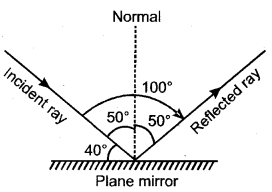A very small head: Convex mirror
A fat body: Concave mirror, and
Legs of normal size: Plane mirror.

Question 19.
Differentiate between virtual image formed by a concave mirror and of a convex mirror.
Plane mirror
The virtual image formed by a concave mirror is always magnified whereas the virtual image formed by a convex mirror is diminished.

Question 20.
The angle between an incident ray and the mirror is 40°.

1. What is the angle of incidence?
2. what is the angle of reflection?
3. what is the total angle through which the ray of light turns?

1. 50°
2. 50°
3. 100°

Question 21.
Why does a convex mirror is said to have a virtual principal focus?
In a convex mirror, a parallel beam after reflection do not actually pass through the focus (F) but it appears to come from the back side of the mirror from focus. So, a convex mirror has a virtual principal focus, which is situated behind the mirror.

Question 22.
What is the value of 0 in the following ray diagram?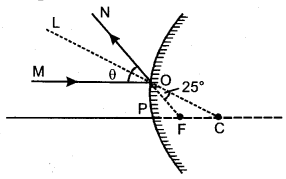Here, ∠MON = ∠MOL + ∠LON = θ
∠LON = ∠FOC = 25° (vetically oppsite angles)
∠LON = ∠MOL = 25° [Angle of incidence = Angle of reflection]
∠MON = 25 + 25 = 50°

Question 23.
Explain why a ray of light passing through the centre of curvature of a concave mirror gets reflected along the same path after reflection.
This is because the angle of incidence is 0°. That is the ray passing through the centre of curvature is incident normally to the mirror. The angle of reflection should also be 0°.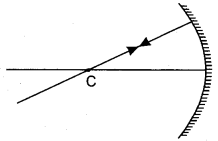Question 24.
What is the nature of the image formed by a concave mirror if the magnification produced by the mirror is + 3?
Virtual and erect.

Question 25.
Between which two points of a concave mirror should an object be placed to obtain a magnification of -3?
Between F and C.

Question 26.
Draw a ray diagram to show the path of the reflected ray corresponding to an incident ray of light parallel to the principal axis of a convex mirror and show the angle of incidence and angle of reflection on it.Question 27.
The outer surface of a hollow sphere of aluminium of radius 50 cm is to be used as a mirror. What will be the focal length of this mirror? Which type of spherical mirror will it provide?
Focal length, f = $$\frac {R}{2}$$ = $$\frac {50}{2}$$ = 25 cm
It will provide a convex mirror.

Question 28.
Which property of concave mirror is utilised for using them as shaving mirrors?
When an object is placed between the pole and focus of concave mirror a magnified, erect and virtual image is obtained.

Question 29.
What is an optically rarer medium?
A medium in which light travels comparatively faster than the other medium is called an optically rarer medium.

Question 30.
What is an optically denser medium?
A medium in which light travels comparatively slower than the other medium is called an optically denser medium.

Question 31.
Define the term refraction of light.
The bending of a ray of light falling obliquely on a surface when it passes from one transparent medium to another is called refraction.

Question 32.
Define the term angle of incidence.
The angle between the incident ray and the normal at the point of incidence is called angle of incidence.

Question 33.
Define the term angle of refraction.
The angle between the refracted ray and the normal at the point of incidence is called angle of refraction.

Question 34.
Define the term refractive index of a medium in terms of speed of light.
Refractive index of a medium is defined as the ratio of speed of light in vacuum to the speed of light in the medium. i.e.,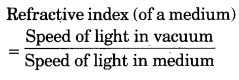Question 35.
What is absolute refractive index?
Refractive index of a medium with respect to vacuum is called absolute refractive index.

Question 36.
Write down the Snell’s law of refraction.
The ratio of sine of angle of incidence to the sine of angle of refraction is a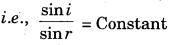The is Snell’s law of refraction.

Question 37.
Refractive index of two material medium X and Y are 1.3 and 1.5 respectively, In which of the two, the light would travel faster?
In medium ‘X’ because of lower value of refractive index.

Question 38.
What is the cause of refraction of light?
Refraction of light takes place when it travels from one medium to another because the speed of light is different in the two media.

Question 39.
What is the relationship between the refractive index of two media?
The refractive index for the light going from medium ‘1’ to medium ‘2’ is equal to the reciprocal of the refractive index for light going from medium ‘2’ to medium ‘1’.Question 40.
In which direction a ray of light bends when it goes from water to glass?
We know that glass is a denser medium than water. Therefore, a ray of light will bend towards the normal when it goes from water to glass.

Question 41.
If refractive indices of water and alcohol are 1.33 and 1.36 respectively, which of the two is optically denser medium?
The refractive index of alcohol is more than water, therefore, alcohol is optically denser medium.

Question 42.
If a light ray IM is incident on the surface AB as shown, identify the correct emergent ray.Here, NQ is parallel to OS. Therefore, NQ is the correct emergent ray.

Question 43.
Why does a ray of light bend when it travels from one medium into another?
When a ray of light travels from one medium to another, its speed changes and this change in speed of light causes the bending of light (refraction of light).

Question 44.
What is a lens?
A lens is a piece of transparent medium bounded by two surfaces of which at least one surface is curved.

Question 45.
Name the point inside a lens such that a ray of light passing through it goes undeviated.
Optical centre.

Question 46.
Name the phenomena on which the working of a lens is based. Answer: The working of a lens is based on the penomenon of refraction of light.

Question 47.
State two examples of phenomenon of refraction of light in everyday life

1. A stick partly immesed in water appears to be bent at the water surface.
2. A pool of water appears less deep than it actually is.

Question 48.
What is meant by power of a lens?
Power of a lens is the degree of convergence or divergence of light rays achieved by a lens, P = $$\frac{1}{f}$$ (where f = focal length).

Question 49.
Give the SI unit of power of lens. State whether the power of a converging lens is positive or negative.
The SI unit of power of a lens is dioptre.
The power of a converging lens is positive as f is +ve.

Question 50.
With the help of convex lens how will you obtain:
(i) Parallel rays,
(ii) Convergent rays and
(iii) Divergent rays from a light source?
Parallel rays. If light source is at first focus of convex lens then after refraction rays become parallel.Convergent Rays. If light source is at infinity then after refraction through the lens they would converge at the focus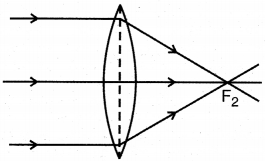Divergent Rays. If light source is between first focus and lens, then after refraction divergent rays are formed.Question 51.
A spherical mirror and a lens have same focal length of – 20 cm. What type of mirror and lens are these?
A concave mirror and concave lens have negative focal length. Hence, both mirror and lens are concave.

Question 52.
An object is placed 80 cm from a converging lens of focal length 25 cm. What is the natrue of the image?
The image is real, inverted and diminished as the object is placed beyond 2F.

Question 53.
What is the power of a combination of lenses?
If a number of lenses are placed in close contact, then the power of the combination of lenses is equal to algebraic sum of the powers of the individual lenses.
P = P + P + P + …….

Question 54.
State one advantage of using combination of lenses in optical instruments instead of a single lens.
The use of a combination of lenses increases the magnification and sharpness of the image.

Question 55.
The magnification of image of an object is positive for a convex lens. What is the nature of the image?
Virtual.

Question 56.
Name the component of white light that has the greatest wavelength.
Red.

Question 57.
How does phenomenon of lateral inversion occurs?
The phenomenon of lateral inversion occurs due to the reflection of light by plane mirror.

Question 58.
Under what condition a lens becomes invisible when placed in a transparent liquid?
This happens when the refractive index of the lens becomes equal to that of the liquid.

Question 59.
Define the term magnification.
The ratio of the height of image to the height of object is called magnification.

### Light Reflection and Refraction Extra Questions Short Answer Type

Question 1.
What possible phenomenon can happen when light falls on a surface?
Any of the following phenomenon may happen:

1. A portion of incident light is reflected back into the first medium.
2. Some portion travels through the second medium along a changed path, known as refraction.
3. The remaining part of the light may be absorbed by the second medium.

Question 2.
List four characteristics of the images formed by plane mirrors?

1. Image formed by a plane mirror is always virtual and erect.
2. The size of the image is equal to that of the object.
3. The image formed is an far behind the mirror as the object is in front of it.
4. The image is laterally inverted.

Question 3.
List four specific characteristics of the images of the objects formed by convex mirrors.

• Virtual
• Erect
• Diminished
• Object distance more than image distance.

Question 4.
An object is placed at a distance of 30 cm in front of a convex mirror of focal length 15 cm. Write four characteristics of the image formed by the mirror.
Virtual, erect, diminished, laterally inverted.

Question 5.
An object is placed at a distance of 40 cm in front of a convex mirror of radius of curvature 40 cm. List four characteristics of the image formed by the mirror…
Virtual, erect, diminished, laterally inverted.

Question 6.
An object is placed at a distance of 12 cm in front of a concave mirror of radius of curvature 30 cm. List four characteristics of the image formed by the mirror.
Virtual, erect, diminished, laterally inverted.

Question 7.
Explain the term lateral inversion.
If an object is placed in front of a plane mirror, then the right side of the object appears to be the left side of the image, and the left side of the object appears to be the right side of its image. This change of sides of an object and its mirror image is called lateral inversion.

Question 8.
In what way is the word AMBULANCE printed in front of the hospital vans? Why is it printed this way?.
The word AMBULANCE on the hospital vans is written in the form of its mirror image as ZONAJUAMA alphabets correctly from his rear-view mirror and make way for it to pass throuh and enable it to reach the hospital quickly.

Question 9.
Why is colour of clear sky blue?
During the day time, sky appear blue. This is because the si∠e of the particles in the atmosphere is smaller than the wavelength of light, so they scatter the light of shorter wave length.

Question 10.
State the laws of refraction of light. If the speed of light in vacuum is 3 108 m’s, find the absolute refractive
index of a medium in which light travels with a speed of 1.4 × 108 m/s.
Laws of refraction of light:
First Law: The incident ray, the refracted ray, and the normal at the point of incidence all lie in the same plane.
Second Law (Snell’s law): The ratio of sine of angle of incidence to the sine of angle retraction is a constant for a given pair of media.= Constant Speed of light in vacuum, c = 3 × 108 m/s
Speed of ight in medium, v = 1.4 × 108 m/s
Speed of light in vacuum 3 × 108 m/s
Absolute refractive index =Question 11.
State two positions in which a concave mirror produces a magnified image of a given object. List two differences between the two images.
A concave mirror produces a magnified image when the object is placed in front of the mirror:

1. between its pole and focus.
2. betweer. the focus and centre of curvature In case
3. the image is virtual and erect, whereas in case
4. the image is real and inverted.

Question 12.
An object is placed at a distance of 30 cm from a concave lens of focal length 15 cm. List four characteristics (nature, position, etc.) of the image formed by the lens.

• Virtual
• Erect
• Diminished
• On the same side as the object.

Question 13.
The linear magnification produced by a spherical mirror is + 3. Analyse this value and state the
(i) type of mirror and
(ii) position of the object with respect to the pole of the mirror. Draw ray diagram to show the formation of image in this case.

• Concave mirror
• Between the pole and focus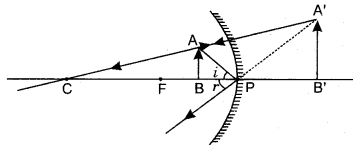Question 14.
Redraw the following diagram on your answer-sheet and show the path of the reflected ray. Also mark the angle of incidence (∠i) and the angle of reflection (∠r) on the diagram.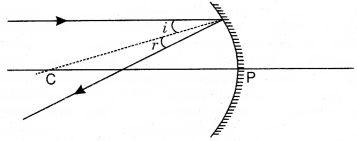Question 15.
What will happen to a ray of light when it falls normally on a surface? Show it diagrammatically.
When a ray of light falls normally on the surface of a medium, then, no bending of light ray occurs. It means the light ray goes straight from one medium to another.

Question 16.
Identify the device used (a spherical mirror or lens) in following cases, when the image formed is virtual Glass and erect in each case.
(a) Object is placed between device and its focus, image formed is enlarged and behind it.
Air (b) Object is placed between the focus and device, image formed is enlarged and on the same side as that of the object.
(c) Object is placed between infinity and device, image formed is diminished and between focus and optical centre on the same side as that of the object.
(d) Object is placed between infinity and device, image formed is diminished and between pole and focus, behind it.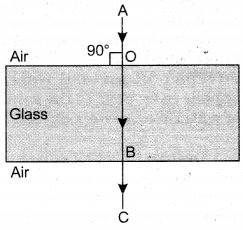(a) Concave mirror
(b) Convex lens
(c) Concave lens
(d) Convex mirror

Question 17.
A convex lens of focal length 20 cm can produce a magnified virtual as well as real image. Is this a correct statement? If yes, where shall the object be placed in each case for obtaining these images?.
Statement is correct if the object is placed within 20 cm from the lens in the first case and between 20 cm and 40 cm in the second case.

Question 18.
How are power and focal length of a lens related? You are provided with two lenses of focal length 20 cm and 40 cm respectively. Which lens will you use to obtain more convergent light?Power of a lens is inversely proportional to its focal length therefore lens having focal length of 20 cm will provide more convergence.

Question 19.
Distinguish between real and virtual image.
Real Image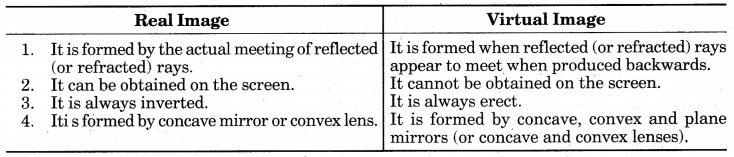Question 20.
Which type of mirror you will use to view the vehicle approaching your car or bike from the rear side? Answer with reasons. Whether the image formed will be real or virtual?
Convex mirrors are commonly used as rear view mirrors is vehicles because they

always give and erect image and have wider field of view as they are curved outward. Image will be virtual.

Question 21.
Explain with the help of a ray diagram, why a pencil partly immersed in water appears to be bent at the water surface.A straight pencil ABC is immersed in water. The portion BC appears to be shorten and bent as BC’. It is due to refraction. Virtual image of C is formed at C”. Thus part BC appears to be BC”. Thus portion of stick under water appears to be P raised and bent at point B.

Question 22.
A pencil when dipped in water in a glass tumbler, appears to be bent at the interface of air and water. Will the pencil appear to be bent to the same extent, if instead of water we use liquids like, kerosene or turpentine. Support your answer with reason.
No. Bending will be different in different liquids since speed of light in the medium depends on the relative refractive index of the medium. A substance having higher refractive index optically denser than another substance having lower refractive index. Thus higher the refractive index of a substance, more it will change the direction of a beam of light passing through it.

Question 23.
How is the refractive index of a medium related to the speed of light? Obtain an expression for refractive index of a medium with respect to another in terms of speed of light in these two media?
The refractive index of the medium nm is given byLet v1 be the speed of light in medium 1 and v, be the speed of light in medium 2. The refractive index of medium 2 with respect to medium 1 is given by the ratio of the speed of light in medium 1 and the speed of light in medium 2.This is usually represented by the symbol n21.
Refraction Class 10 Extra Questions with Answers Science Chapter 10 20″>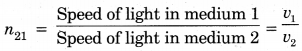Question 24.
The mirage is seen in deserts. Explain the causes.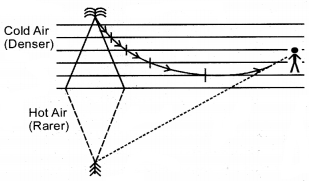The Mirage: On a hot summer day, a traveller in a desert region often sees a water lake with the trees and sky reflected in it, a short distance ahead of him. As he goes ahead, the lake disapp-ears and he finds only a tree in the hot sand. This optical illusion is called mirage.

Mirage can even be seen on a road. On a hot summer day the road at a distance appears to be covered with water, and we can see the reflection of sky and other objects from a distance. But as we approach the place where the water appeared to be, we find that there in no water at all, and that it was just an illusion.

The phenomenon of mirage is produced by the total internal reflection of light which takes place when light passes from optically denser cold air to optically rarer hot air in the atmosphere.

Question 25.
Sudha finds out that the sharp image of the window pane of her science laboratory is formed at a distance of 15 cm from the lens. She now tries to focus the building visible to her outside the window instead of the window pane without disturbing the lens. In which direction will she move the screen to obtain a sharp image of the building? What is the approximate focal length of this lens?
Sudha should move the screen towards the lens so as to obtain a clear image of the building. The approximate focal length of this lens will be 15 cm. The rays of light coming from distant object such as a tree (or a distant building or electricity pole) can be considered to be parallel to each other. When parallel rays of light are incident on a convex lens, the rays, after refraction, converge at focus on the other side of the lens.

### Light Reflection and Refraction Extra Questions Long Answer Type

Question 1.
What do you understand by power of a lens? What is the relation between power of lens and its focal length? Write unit of power.
The power of a lens is defined as the reciprocal of its focal length in metre.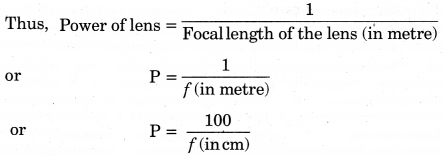The S.I. unit of the power of a lens is ‘diopter’, which is denoted by the letter D. One dioptre is the power of a lens whose focal length is one metre. Power of a convex lens is positive whereas that of a concave lens is negative.

Question 2.
Draw a ray diagram for the image formed by a convex lens when object is kept at: (i) 2f, (ii) F1, (iii) between F and optical centre, (iv) infinity and (v) beyond 2F
(i) When the object is placed at 2F. In this case, the image is formed at 2F on the right hand side of the lens. The image is real, inverted and equal in size with the object.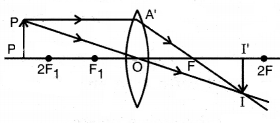(ii) When the object is placed at the focus. In this case, the image is formed at infinity, the nature of image is real, inverted and very much enlarged in size.(iii) When the object is placed between the focus and optical centre of the lens. In this situation, the image is formed on the same side as object (i.e., on the left hand side of the lens). The image is virtual, erect and enlarged.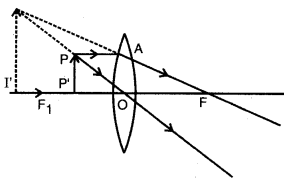(iv) When the object is placed at infinity. Light rays coming from infinity are always parallel to each other and thus, after refraction through the lens they would converge at the focus (prin-cipal focus). Therefore, the image of the object is obtained at the focus and it is real, inverted and highly diminished.(v) When the object is placed beyond 2F. In this case the image is formed between Fand 2F on the right hand side of the lens. The image is real, inverted and diminished.Question 3.
(a) Explain total internal reflection on light. Mention condi-tion for total internal reflection.
(b) Explain refraction of light through a prism.
Or
Define critical angle. Establish the relation between the critical angle and refractive index of the medium.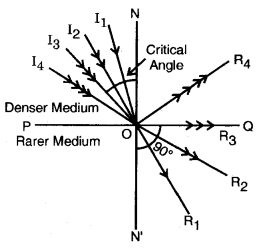(a) Total Internal Reflection of Light. In the fig. PQ is the separating surface of a rarer and denser medium. A ray of light I10 enters from a denser medium to a rarer medium, it bends away from the normal in the direction OR1.

Therefore angle of refraction ∠R1ON’ is greater than angle of incidence ∠I1ON. As the angle of incidence is gradually increased the corresponding angle of refraction also increases. For a certain angle of incidence in the denser medi-um angle of refraction in the rarer medium becomes 90°. This particular angle of incidence in the denser medium is called critical angle.

Therefore ∠I3ON is critical angle (C).
If the angle of incidence is further increased beyond the critical angle then rayinstead of being
refracted gets totally internally reflected according to laws of reflection. This phenomenon is called total internal reflection. In the figure incident ray I4O gets internally reflected in the direction OR4.

Conditions for total internal reflection:

1. The ray must pass from a denser to a rarer medium.
2. The angle of incidence in the denser medium must be greater than the critical angle for that particular medium.

If refractive index of rarer medium with respect to denser medium, is jn, and for these medium critical angle is C then,
for i = C, r = 90°
∴ From Snell’s law,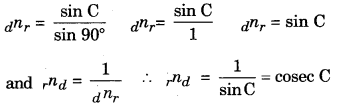(b) Refraction of Light through a Prism. In the Fig., a ray of light PQ is incident on the refracting surface AB of the prism ABC. If medium around prism is rarer in comparison to the prism then ray of light after refraction bends towards the normal. Then again it gets refracted by the refracting surface AC and emerges out in the direction RS (now it bends away from the normal as it is coming from a denser to rarer medium). So, we see that prism deviates light from its path.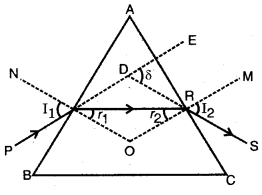Angle between incident ray and emergent ray p (produced backward) is called angle of deviation. It is B represented by letter g. The value of angle of deviation is dependent on the angle of incidence, the angle of the prism and the material of the prism. For a particular angle of incidence, angle of deviation is least. This is called angle of minimum deviation. If angle of prism is A and its angle of minimum deviation is Om, the refractive index of the prism is given by the formula,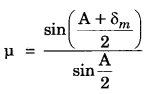### Light Reflection and Refraction Extra Questions Numerical Type

Question 1.
The image of a candle flame placed at a distance of 30 cm from a mirror is formed on a screen placed in front of the mirror at a distance of 60 cm from its pole. What is the nature of the mirror? Find its focal length. If the height of the flame is 2.4 cm, find the height of its image. State whether the image formed is erect or inverted.
The mirror is concave h1 = + 2.4 cm u = – 30 cm υ = -60 cm f = ?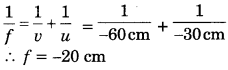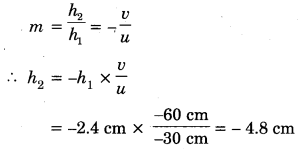-ve sign of h, image size) indicates that the image is inverted

Question 2.
Find the size, nature and position of image formed when an object of size 1 cm is placed at a distance of 15 cm from a concave mirror of focal length 10 cm.
Object distance, u = -15 cm
Focal length, f = – 10 cm
Object size, h = 1 cm
Image distance, υ = ?
Image size, h’ = ?

(i) Position of imageThe image is formed at a distance 30 cm on the side of the object. Negative sign indicates that object and image are on the same side.
(ii) Nature of image: The image is in front of the mirror, its nature is real and inverted.
(iii) Size of image: From the expression for magnifcation,Image size, h’ = -2 cm
The image formed has size 2 cm and negative sign means inverted and real.

Question 3.
An object 2 cm high is placed at a distance of 16 cm from a concave mirror which produces a real image 3 cm high.
(i) Find the position of the image
(ii) What is the focal length of mirror?
Object height, h = + 2 cm
Image height, h = -3 cm (real image hence inverted)
Object distance, u = -16 cm
Image distance, υ = ?
Focal length, f = ?
(i) Position of image
From the expression for magnification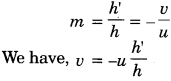Putting values, we get v = -(-16) × $$\frac{-3}{2}$$
v = -24 cm
The image is formed at distance of 24 cm in front of the mirror (negative sign means object and image are on the same side).

(ii) Focal length of mirrorQuestion 4.
A 2 cm high object is placed at a distance of 32 cm from a concave mirror. The image is real, inverted and 3 cm in size. Find the focal length of the mirror and the position where the image is formed?
Given: Object height, ho= 2 cm, Image height, he = -3 cm, Object distance, u = -32 cm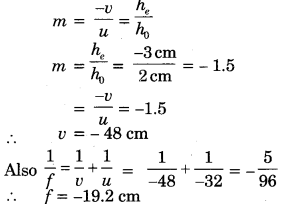∴ Focal length of concave mirror is 19.2 cm and image is formed 48 cm in front of it.

Question 5.
An object 4 cm in height, is placed at 15 cm in front of a concave mirror of focal length 10 cm. At what distance from the mirror should a screen be placed to obtain a sharp image of the object. Calculate the height of the image.
h1 3 + 4 cm f = -10 cm u = -15 cm v = ? h2 = ?Question 6.
A convex mirror with radius of curvature 5 metre is attached with a motor cycle to see the objects behind the motorcycle. Describe the position, nature and size of the image, formed by this mirror of another motorcycle coming from behind at a distance of 5 metre.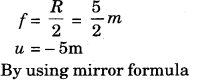Image is virtual and has a size of one-third of object.

Question 7.
The image of an object is formed on the object itself placed at 36 cm from the pole on the principal axis of a concave mirror. Calculate the focal length of the mirror and linear magnification:
Given: u = – 36 cm, v = -36 cm, f = ?, m = ?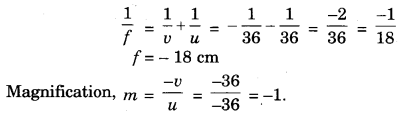Question 8.
A truck uses a convex mirror as a viewfinder whose radius of curvature is 2.0 m.  A maruti car is coming behind the truck at a distance of 10 m. What will be the position of the image of the car and size of the image of the car when observed by the driver of the truck through the convex mirror?
For convex mirror, we have given, u = -10 m, R = 2.0 m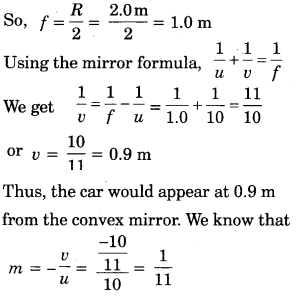Thus, size of the image of the car will be a fraction of $$\frac {1}{11}$$ the actual size of the car through the convex mirror.

Question 9.
If the angle of incidence
(i) for a light ray in air be 45° and the angle of refraction (r) in glass be 30°, find refractive index of glass with respect to air.
Refractive index of glass,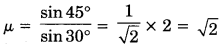Question 10.
Refractive index of water with respect to air is 1.33. What is the value of refractive index of air with respect to water?
Here,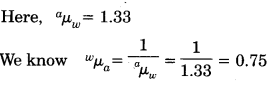Question 11.
The absolute refractive indices of glass and water are 3/2 and 4/3 respectively. If the speed of light in glass is 2 × 108 m/s, calculate the speed of light in (i) vacuum, (ii) water.Speed of light in water Speed of light in water = 2.25 × 108 m/s

Question 12.
Refractive index of water with respect to air is 4/3 and glass is 3/2. What is the refractive index of glass with respect to water?
When there are three media air, water and glass,
We have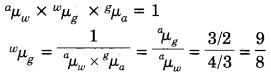∴ Refractive index of glass with respect to water is 9/8.

Question 13.
Refractive indices of water and benzene with respect to air are 1.33 and 1.50 respectively. Calculate the refractive index of benzene with respect to water.
For air, water and benzene,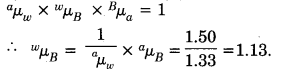Question 14.
Refractive index of diamond with respect to glass is 1.6 and absolute refractive index of glass is 1.5. Find out the absolute refractive index of diamond.
For air, glass and diamond,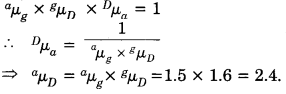Question 15.
The absolute refractive indices of two media ‘A’ and ‘B’ are 2.0 and 1.5 respectively. If the speed of light in medium ‘B’ is 2 × 108 m/s, calculate the speed of light in:
(i) Vacuum
(ii) medium ‘A’.
nA = 2.0; nB = 1.5; speed of light in medium B, UB = 2 × 108 m/sQuestion 16.
An object is placed perpendicular to the principal axis of a convex lens of focal length 8 cm. The distance of the object from the lens is 12 cm. Find the position and nature of the image.
u = -12 cm, f = + 8 cm, ν = ?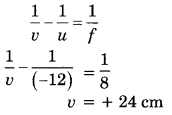The image is real and inverted.

Question 17.
Calculate the position of an image of an object placed 30 cm away from a concave lens of focal length 15 cm. Also calculate the magnifying power of the lens.
Given: f = -15 cm, u = – 30 cm, ν = ?, m = ?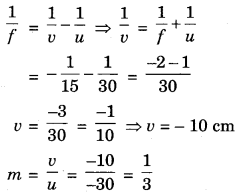Question 18.
An object 3 cm high is placed 20 cm from convex lens of focal length 12 cm. Find the nature, position and height of the image.
Since lens is convex, thereforef is positive.
Given: u = – 20 cm, f = + 12 cm, h = 3 cm, ν =? h’ = ?
Using lens formula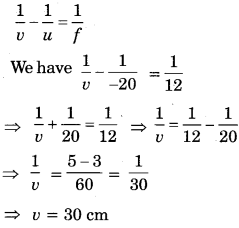Since ‘ν’is positive, the image is located on the other side of the lens.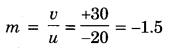Since m is negative and greater than 1, the image is real, inverted and larger than the object.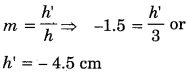Thus the image is 30 cm from the convex lens, located on the other side of the lens. It is real, inverted and 4.5 cm high.

Question 19.
A real image, size of the object is formed 18 cm from a lens. Calculate the focal length of the lens.
Since the image is real and diminished, the lens must be convex and the object must be placed beyond 2F
Given: ν = + 18 cmQuestion 20.
An object kept at a distance of 60 cm from a lens gives a virtual image at a distance of 20 cm over the same side of the lens. What is the focal length of the lens? Is the lens converging or diverging?
Here, u = -60 cm, ν = – 20 cm
From lens formula,The focal length of the lens is 30 cm. The -ve sign shows that the lens is diverging.

Question 21.
If focal length of a convex lens is 20 cm. Find the power of lens.
f = 20 cm, P = ?
P = $$\frac{100}{f}$$ = $$\frac{100}{20}$$ = + 5D

Question 22.
A student uses a lens of focal length 50 cm and another of -50 cm. What is the nature of the lens and its power used by each of them?
Case I: When f =50 cm = 0.5 m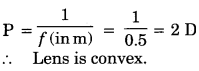∴ Lens is concave.

Question 23.
Two thin lenses of focal lengths +10 cm and -5 cm are kept in contact. What is the focal length and power of the combination?
Here, fi = + 10 cm, f = -5 cm,
∴ Focal length of the combiantion of lenses is given by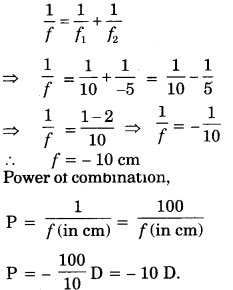Question 24.
An object 5 cm in length is held 25 cm away from a converging lens of focal length 10 cm. Draw the ray diagram and find the position, size and the nature of the image formed.
O = 5 cm, u = -25 cm, f = + 10 cm, I = ?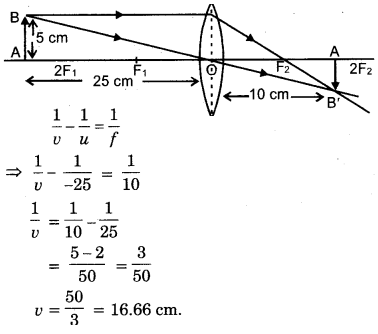Image is for 16.66 cm (ray 16.7 cm) from the lens on other side, i.e., behind the lens. Image is real and inverted.Image is inverted and a height of 3.33 cm.

Question 25.
A concave lens of focal length 15 cm for an image 10 cm from the lens. How far is the object placed from the lens? Draw the ray diagram.
f = – 15 cm, ν = -10 cm, u = ?Object is placed at a distance of 30 cm from concave lens.

Question 26.
An object is placed at a distance of 10 cm from a convex mirror of focal length 15 cm. Find the position and nature of the image.
f = 15 cm, u = – 10 cm, ν = ?Image is formed 6 cm behind the mirror. Image is virtual and erect.

Question 27.
A 5.0 cm long object is placed at a distance of 20 cm from ‘a convex lens of focal length 10 cm. Object is placed normal to the principle axis of the lens. Find out nature, position and si∠e of the image.
Given: u = – 20 cm, f = 10 cm, O = 5 cm, ν = ?, I = ?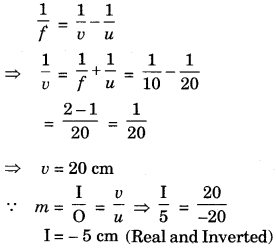Question 28.
An object of size 7.0 cm is placed at 27 cm in front of a concave mirror of focal length 18 cm. At what distance from the mirror should a screen be placed, so that a sharp focused image can be obtained? Find the size and the nature of the image.
O = 5.0 cm, u = -27 cm, f = -18 cm ν = ? I = ?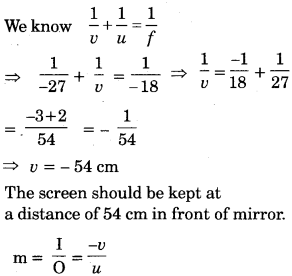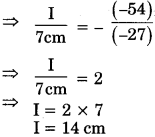Height of image is 14 cm, the image is real and inverted.

Question 29.
Find the focal length of a lens of power -2.0 D. What type of lens is this?The lens is concave lens.

Question 30.
A doctor has prescribed a corrective lens of power + 1.5 D. Find the focal length of the lens. Is the prescribed lens diverging or converging?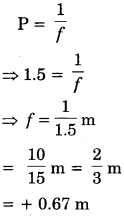Focal length of the lens is + 0.67 m. The prescribed lens is converging in nature.

Question 31.
Sunlight is incident on a concave mirror, parallel to its principal axis. The image is formed at a distance of 20 cm from the pole. Find the radius of curvature of the mirror.
Rays from the sun incident parallel to the principal axis meet at the focus after reflection by the mirror. Thus, the focal length of the mirror f = 20 cm. . Therefore, radius of curvature R = 2f = 2 × 20 = 40 cm.

Question 32.
A concave lens has focal length 15 cm. If image is formed at a distance 10 cm from the lens, find the position of object. Calculate the magnification produced by the lens also.
Given: f = -15 cm, v = –10 cm, u = ?, m = ?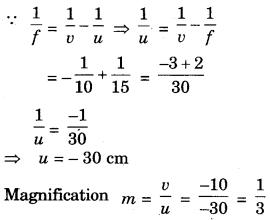Question 33.
Find the position of the image of an object placed at a distance of 25 cm in front of a concave mirror of focal length 50 cm.
Here
u= -25 cm
and f = -50 cm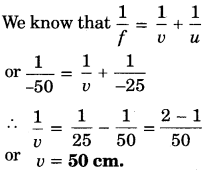Since ν is positive, therefore image is formed behind the mirror at a distance of 50 cm from the mirror.

Question 34.
A 1.5 cm high object is placed at a distance of 25 cm from a concave mirror. A real image is formed at a distance of 75 cm from the mirror. Calculate the focal length of the mirror and the size of the image.
Since the image is real, it is formed in the same side of the mirror. Therefore, u = – 25 cm, ν = – 75 cm, f = ?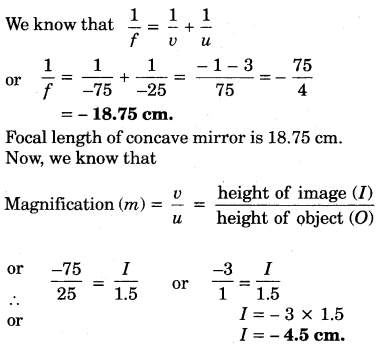The image is inverted and 4.5 cm high.

Question 35.
A 2 cm high object is placed at a distance of 20 cm from a concave mirror. The image is real, inverted and 3 cm in size. Find the focal length of the mirror and the position of the image.
We know that,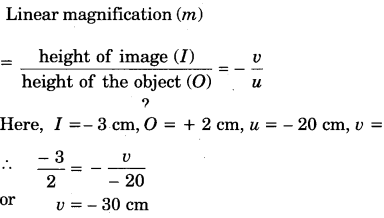Thus, image is formed on the same side and 30 cm away from the mirror.Thus, focal length of concave mirror is 12 cm.

Question 36.
Refractive indices of water and glass with respect to air are $$\frac{4}{3}$$ and $$\frac{3}{2}$$ respectively. Find the refractive index of glass with respect to water.
Given that: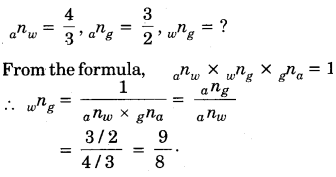Question 37.
Speed of light in vacuum is 3.0 × 108 m/s. Calculate the speed of light in water. (Refractive index of water = 4/3).
Solution.
Given that: C = 3.0 × 108 m/s, n = $$\frac{4}{3}$$, ν = ?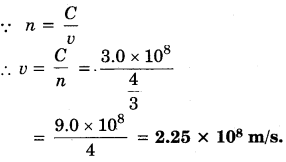Question 38.
Refractive index of water is $$\frac{4}{3}$$. Find the critical angle of water. [sin 48° 36′ = 0.75].
Given that:
n = $$\frac{4}{3}$$, C = ?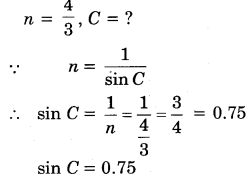sin C = sin 48°36′
C = 48°36′

Question 39.
The distance of object from a lens is 15 cm. The virtual image formed four times of the size of object. Find the distance of image and focal length of lens.
Solution.
Given that: u = -15 cm, m = + 4, ν = ?, f = ?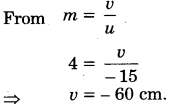Hence, image will from 60 cm distance from lens in the object side.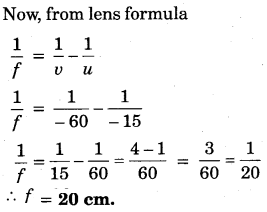Question 40.
The image of an object formed by a convex lens is 3 times of object on screen. If the positions of object and screen are interchanged, then what will be its magnification in this condition?On interchanging the positions of object and screen, ν and u are also changed.Question 41.
The focal length of a convex lens is 30 cm and an object is kept at 10 cm from lens. What will be the distance and nature of image?
Solution.
Given that: f = 30 cm, u = – 10 cm, ν = ?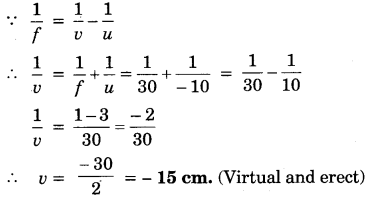Question 42.
The power of a lens is + 2D. Calculate its focal length. Is this lens convergent or divergent?
f = $$\frac{100}{P}$$ $$\frac{100}{2}$$ = 50 cm (Convergent)